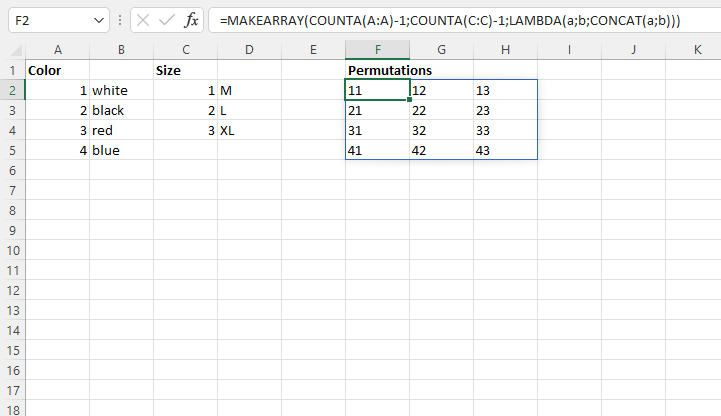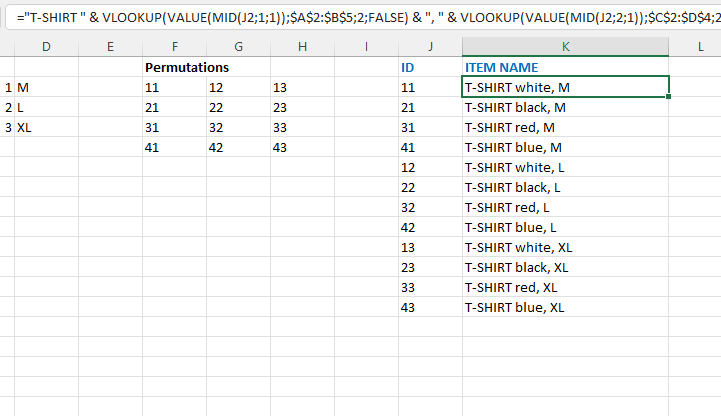# Generating item codes

It is a common practice in domestic retail outlets to keep a large number of items under one code. In fact, it is one item, but its additional attributes are not taken into account, such as: color, size, design, season … Consequently, the stocks are incorrect. How to form item codes in such a way that, within the code itself, all its attributes are taken into account? We will use permutations!

Let’s use permutations to generate codes for T-shirts of different colors and sizes. We will start with two tables with color and size labels, and to generate permutations you need to use the MAKEARRAY function. Let’s write the formula:

=MAKEARRAY(COUNTA(A:A)-1,COUNTA(C:C)-1,LAMBDA(a,b,CONCAT(a,b)))

The formula counts the contents of the columns that contain the color and size codes, so it passes this information to the function. We reduced the number by one to ignore the header of each of the columns. The result of this formula is a table with all color and size permutations.The next step is to transform the matrix into a column. We will achieve this using the formula:

=OFFSET(\$F\$2:\$H\$5,MOD(ROW()-ROW(\$F\$2),ROWS(\$F\$2#)),
TRUNC((ROW()-ROW(\$F\$2))/ROWS(\$F\$2:\$H\$5)),1,1)

Once we have entered the formula we will copy it into as many cells as the matrix elements it contains. We will use this column for item codes. Then you need to write a formula to generate the name:

=”T-SHIRT ” & VLOOKUP(VALUE(MID(J2,1,1)),\$A\$2:\$B\$5,2,FALSE)
& “, ” & VLOOKUP(VALUE(MID(J2,2,1)),\$C\$2:\$D\$4,2,FALSE)The result is a table with codes and T-shirt names. The table generated in this way can be easily downloaded to the ERP, WMS or other business information system in order to record the items on the basis of which we monitor inventory.